Home > INT3 > Chapter 7 > Lesson 7.2.3 > Problem7-81

7-81.
1.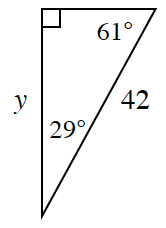Eugene wants to use the cosine ratio to calculate y in this triangle. Homework Help ✎

1. Which angle should he use to write an equation and solve for y using the cosine ratio? Why?

2. Write an equation and solve for y using cosine.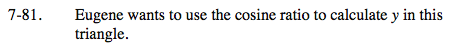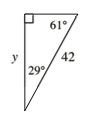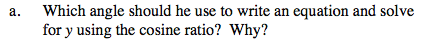He'll need the adjacent side and the hypotenuse. To which angle is y adjacent?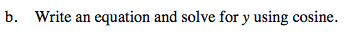$\cos \left(29\degree \right)=\frac{y}{42}$